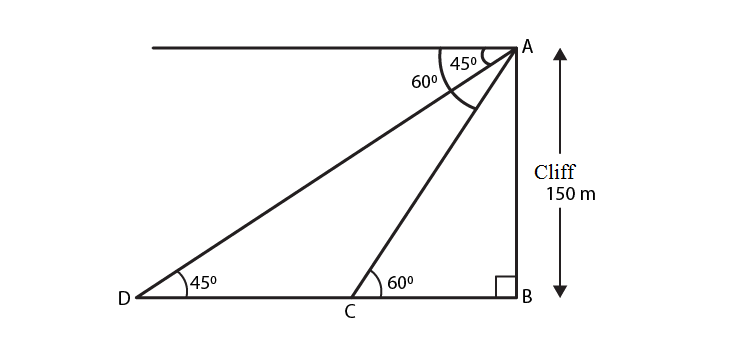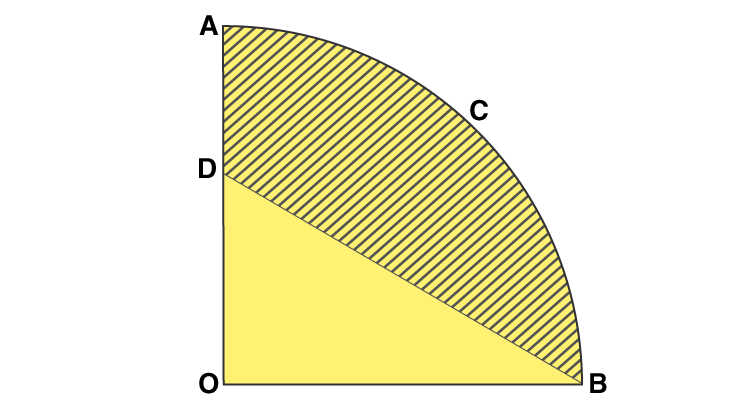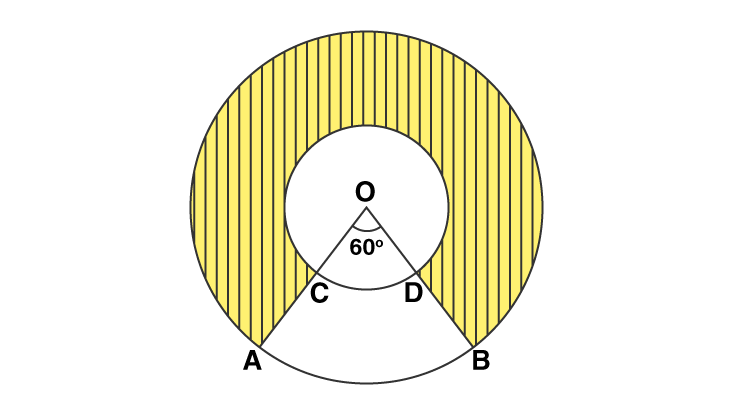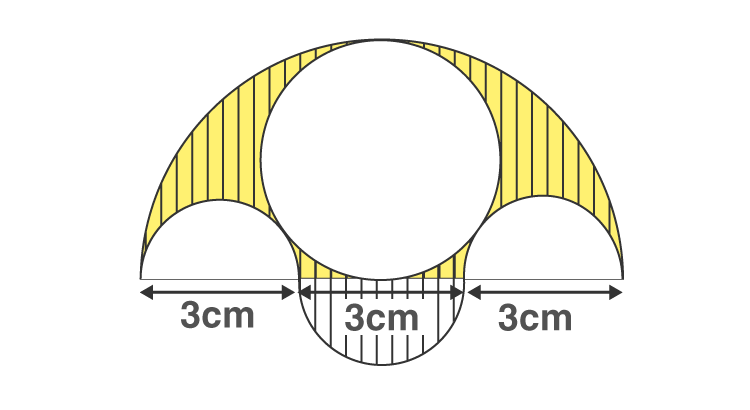# CBSE Class 10 Maths Board Exam: Important 3 marks questions

In the CBSE examination, Mathematics is one of the subjects for which students feel that they are enough prepared and still not confident even after covering the syllabus as the subject requires proper understanding to excel in an examination. For students planning to choose their future career in Engineering, it is pretty essential to do extensively great on the subject.

Class 10 maths paper contains 1, 2, 3 & 4 marks questions, whereas one-third of the paper comprises 3 marks questions. Thus, if one wishes to excel in the examination, a good practice of 3 marks questions is essential. Hence, we at BYJU’S provide class 10 important 3 marks questions from an exam perspective to help students score good marks.

Also, access: Important Questions for Class 10 Maths

Students preparing for CBSE Class 10 Maths Board Examination are advised to practice the questions provided below and verify solutions. Also, get the additional questions provided below to improve your problem solving skills.

Important 4 Marks Questions and Solutions for Class 12 Maths Board are as follows-

Question 1- If mth term of an A.P. is

$$\begin{array}{l}\frac{1}{n}\end{array}$$
and nth term is
$$\begin{array}{l}\frac{1}{m}\end{array}$$
, then find the sum of its first mn terms.

Solution:

Let a be the first term and d be the common difference of an AP.

Given,

am = 1/n

i.e. a + (m – 1)d = 1/n

n[a + (m – 1)d] = 1

an + mnd – nd = 1….(i)

And

an = 1/m

a + (n – 1)d = 1/m

m[a + (n – 1)d] = 1

am + mnd – md = 1….(ii)

From (i) and (ii),

an + mnd – nd = am + mnd – md

an – am = nd – md

a(n – m) = (n – m)d

a = d

Substituting a = d in equation (i),

dn + mnd – nd = 1

mnd = 1

d = 1/mn

Therefore, a = 1/mn

Sum of first mn terms is:

Smn = (mn/2)[2(1/mn) + (mn – 1)(1/mn)]

= (mn/2)[(2/mn) + (mn – 1)/mn]

= (mn/2mn)[2 + mn – 1]

= (1/2)(mn + 1)

Hence, the sum of first mn terms = (mn + )/2.

Question 2- A moving boat is observed from the top of a 150 m high cliff moving away from the cliff. The angle of depression of the boat changes from

$$\begin{array}{l}60^{\circ}\end{array}$$
to
$$\begin{array}{l}45^{\circ}\end{array}$$
in 2 minutes. Find the speed of the boat in m/h.

Solution:

Let AB be the height of the cliff.

AB = 150 m

C and D be the position of a boat.Boat is moved from C to D in 2 minutes = 2/60 hr = 1/30 hr

In right triangle ABC,

tan 60° = AB/BC

√3 = 150/BC

BC = 150/√3 m

In right triangle ABD,

1 = 150/(BC + CD)

(150/√3) + CD = 150

CD = 150 – (150/√3)

CD = (150√3 – 150)/√3

= 150(√3 – 1)/√3 m

Distance CD = Speed × time

150(√3 – 1)/√3 = Speed × (1/30)

Speed = 30 × [150(√3 – 1)/√3]

= 1500√3(√3 – 1) m/h

Question 3- Two different dice are thrown together. Find the probability that the numbers obtained

(i) have a sum less than 7

(ii) have a product less than 16

(iii) is a doublet of odd numbers.

Solution:

Given,

Two different dice are thrown together.

S = {(1, 1), (1, 2), (1, 3), (1, 4), (1, 5), (1, 6)

(2, 1), (2, 2), (2, 3), (2, 4), (2, 5), (2, 6)

(3, 1), (3, 2), (3, 3), (3, 4), (3, 5), (3, 6)

(4, 1), (4, 2), (4, 3), (4, 4), (4, 5), (4, 6)

(5, 1), (5, 2), (5, 3), (5, 4), (5, 5), (5, 6)

(6, 1), (6, 2), (6, 3), (6, 4), (6, 5), (6, 6)}

Total number of outcomes = n(S) = 36

(i) have a sum less than 7

Let E1 be the event of getting the numbers on dice whose sum is less than 7.

E1 = {(1, 1), (1, 2), (1, 3), (1, 4), (1, 5), (2, 1), (2, 2), (2, 3), (2, 4), (3, 1), (3, 2), (3, 3), (4, 1), (4, 2),(5, 1)}

n(E1) = 15

P(E1) = n(E1)/n(S) = 15/36 = 5/12

(ii) have a product less than 16

Let E2 be the event of getting the numbers on dice whose product is less than 16.

E2 = {1, 1), (1, 2), (1, 3), (1, 4), (1, 5), (1, 6) (2, 1), (2, 2), (2, 3), (2, 4), (2, 5), (2, 6) (3, 1), (3, 2), (3, 3), (3, 4), (3, 5), (4, 1), (4, 2), (4, 3), (5, 1), (5, 2), (5, 3), (6, 1), (6, 2)}

n(E2) = 25

P(E2) = n(E2)/n(S) = 25/36

(iii) is a doublet of odd numbers.

Let E3 be the event of getting a doublet of odd numbers.

E3 = {(1, 1), (3, 3), (5, 5)}

P(E3) = n(E3)/n(S) = 3/36 = 1/12

Question 4- The area of a triangle is 5 sq units. Two of its vertices are (2,1) and (3,-2). If the third vertex is

$$\begin{array}{l}\left ( \frac{7}{2},y \right )\end{array}$$
, then find the value of y.

Solution:

Let A, B, and C be the vertices of a triangle.

A(2, 1) = (x1, y1)

B(3, -2) = (x2, y2)

C(7/2, y) = (x3, y3)

Given,

Area of the triangle is 5 sq.units.

(1/2)|x1(y2 – y3) + x2(y3 – y1) + x3(y1 – y2)| = 5

(1/2)|2(-2 – y) + 3(y – 1) + (7/2)(1 + 2)| = 5

|-4 – 2y + 3y – 3 + (7/2)(3)| = 10

y – 7 + (21/2) = 10

y = 10 + 7 – (21/2)

y = 17 – (21/2)

y = (34 – 21)/2

y = 13/2

Therefore, the value of y is 13/2.

Question 5- The

$$\begin{array}{l}\frac{3}{4}\end{array}$$
th part of a conical vessel of internal radius 5 cm and height 24 cm is full of water. The water is emptied into a cylindrical vessel with an internal radius of 10 cm. Find the height of water in the cylindrical vessel.

Solution:

Given,

Height of conical vessel = h = 24 cm

Radius of conical vessel = r = 5 cm

Volume of water = (3/4) × Volume of conical vessel

= (3/4) × (1/3)πr2h

= (3/4) × (1/3) × (π × 5 × 5 × 24)

= 150π cm3

Let H be the height of a cylindrical vessel.

Radius of cylindrical vessel = R = 10 cm {given}

From the given,

Volume of cylindrical vessel = Volume of water

πR2H = 150π

R2H = 150

10 × 10 × H = 150

H = 1.5 cm

Therefore, the height of a cylindrical vessel.

Question 6- In the given figure, OACB is a quadrant of a circle with centre O and radius 3.5 cm. If OD = 2 cm, find the area of the shaded region.Solution:

Given,

= (1/4) × (22/7) × 3.5 × 3.5

= 9.625 cm2

Area of triangle BOD = (1/2) × OB × OD

= (1/2) × 3.5 × 2

= 3.5 cm2

Area of the shaded region = Area of quadrant – Area of triangle BOD

= 9.625 – 3.5

= 6.125 cm2

Question 7- If the equation

$$\begin{array}{l}(1+m^{2})x^{2} + 2mcx + c^{2} – a^{2} = 0\end{array}$$
has equal roots then show that
$$\begin{array}{l}c^{2} = a^{2}(1+m^{2})\end{array}$$
.

Solution:

Given,

(1 + m2)x2 + 2mcx + c2 – a2 = 0

Comparing with the standard form of a quadratic equation ax2 + bx + c = 0,

a = (1 + m2)

b = 2mc

c = (c2 – a2)

Given that the quadratic equation has equal roots.

So, b2 – 4ac = 0 {determinant is equal to 0}

(2mc)2 – 4(1 + m2)(c2 – a2) = 0

4m2c2 – 4(c2 – a2 + m2c2 – m2a2) = 0

4m2c2 – 4c2 + 4a2 – 4m2c2 + 4m2a2 = 0

4c2 = 4a2m2 + 4a2

4c2 = 4a2(m2 + 1)

c2 = a2(1 + m2)

Question 8- Find the sum of n terms of the series

$$\begin{array}{l}\left ( 4-\frac{1}{n} \right ) + \left ( 4-\frac{2}{n} \right ) + \left ( 4-\frac{3}{n} \right ) + ……..\end{array}$$

Solution:

Given series:

[4 – (1/n)] + [4 – (2/n)] + [4 – (3/n)] + …. up to n terms

= (4 + 4 + 4 + ….. up to n terms) – [(1/n) + (2/n) + (3/n) + …. up to n terms]

= 4n – (1/n)[1 + 2 + 3 + …. up to n terms]

= 4n – (1/n)[n(n + 1)/2]       {sum of first n natural numbers = n(n + 1)/2}

= 4n – (n + 1)/2

= (8n – n – 1)/2

= (7n – 1)/2

Therefore, the sum of the given series is (7n – 1)/2.

Question 9- Prove that: (sec A – cos A). (cot A + tan A) = tan A. sec A

Solution:

LHS = (sec A – cos A)(cot A + tan A)

= [(1/cos A) – cos A] [(cos A/sin A) + (sin A/cos A)]

= [(1 – cos2A)/cos A] [(cos2A + sin2A)/ (sin A cos A)]

= (sin2A/ cos A) [1/(sin A cos A)]

= sin A/(cos A cos A)

= (sin A/cos A) (1/cos A)

= tan A sec A

= RHS

Thus, (sec A – cos A). (cot A + tan A) = tan A. sec A

Hence proved.

Question 10- The following distribution shows the daily pocket allowance of children of a locality. The mean pocket allowance is Rs 18. Find the missing frequency f.

 Daily pocket allowance (in Rs.) 11 – 13 13 – 15 15 – 17 17 – 19 19 – 21 21 – 23 23 – 25 Number of children 7 6 9 13 f 5 4

Solution:

 Class interval Number of children (fi) Mid-point (xi) fixi 11 – 13 7 12 84 13 – 15 6 14 84 15 – 17 9 16 144 17 – 19 13 18 234 19 – 21 f 20 20f 21 – 23 5 22 110 23 – 25 4 24 96 Total ∑fi = 44 + f ∑fixi = 752 + 20f

Mean = x̄ = ∑fixi /∑fi

= (752 + 20f)/(44 + f)

Given that, (752 + 20f)/(44 + f) = 18

752 + 20f = 18(44 + f)

752 + 20f = 792 + 18f

20f – 18f = 792 – 752

2f = 40

f = 20

Therefore, the missing frequency = f = 20

### CBSE Class 10 Maths 3 Marks Important Questions for Practice

1. Two tangents TP and TQ are drawn to a circle with centre O from an external point T. Prove that
$$\begin{array}{l}\angle PTQ = 2 \angle OPQ\end{array}$$
2. In a game, a one-rupee coin is tossed three times and the result is recorded every time. Haneef will win if he gets at least two heads. Calculate Haneef’s probability of losing the game.
3. From a point of a ground, the angles of elevation of the bottom and top of a communication tower fixed on the top of a 20 m high building are
$$\begin{array}{l}45^{\circ}\end{array}$$
and
$$\begin{array}{l}60^{\circ}\end{array}$$
respectively. Find the height of the tower. (Take
$$\begin{array}{l}\sqrt{3} = 1.732\end{array}$$
).
4. Show that
$$\begin{array}{l}\Delta ABC\end{array}$$
, where A(-2,0), B(2,0), C(0,2) and
$$\begin{array}{l}\Delta PQR\end{array}$$
where P(-4,0), Q(4,0), R(0,4) are similar triangles.
5. The mid-points of the side BC, CA, and AB of a
$$\begin{array}{l}\Delta ABC\end{array}$$
are D(3,4), E(8,9) and F(6,7) respectively. Find the coordinates of the vertices of the triangle.
6. Water is flowing into a canal, 6 m wide and 1.5 m deep, at the rate of 10km/hr. How much area will it irrigate in 30 minutes, if 8 cm of standing water is required for irrigation?
7. A solid metallic sphere of diameter 21 cm is melted and recast into a number of smaller cones, each of radius 4.5 cm and height 3 cm. Find the number of cones so formed.
8. A hemisphere of maximum possible diameter is placed over a cuboidal block of side 7 cm. Find the surface area of the solid so formed.
9. If the sixth term of an A.P. is zero, then show that its 33rd term is three times its 15th term.
10. Show that
$$\begin{array}{l}x = -\frac{bc}{ad}\end{array}$$
is a solution of the quadratic equation
$$\begin{array}{l}ad^{2}\left ( \frac{ax}{b} + \frac{2c}{d}\right )x + bc^{2} = 0\end{array}$$
11. In the given figure, two concentric circles with centre O have radii 21 cm and 42 cm. If
$$\begin{array}{l}\angle AOB = 60^{\circ}\end{array}$$
, find the area of the shaded region.12. Three semicircles each of diameter 3 cm, a circle of diameter 4.5 cm and a semicircle of radius 4.5 cm are drawn in the given figure. Find the area of the shaded region.13. If the roots of the equation
$$\begin{array}{l}(c^{2}- ab) x^{2}- 2(a^{2}-bc)x – ac = 0\end{array}$$
in x are equal, then show that either a = 0 or
$$\begin{array}{l}a^{3}+b^{3}+ c^{3} = 3abc\end{array}$$

To practice more questions, students must solve the CBSE board sample papers for class 10. Stay tuned for further updated on CBSE and other competitive exams. To access interactive Maths and Science videos download BYJU’S app.